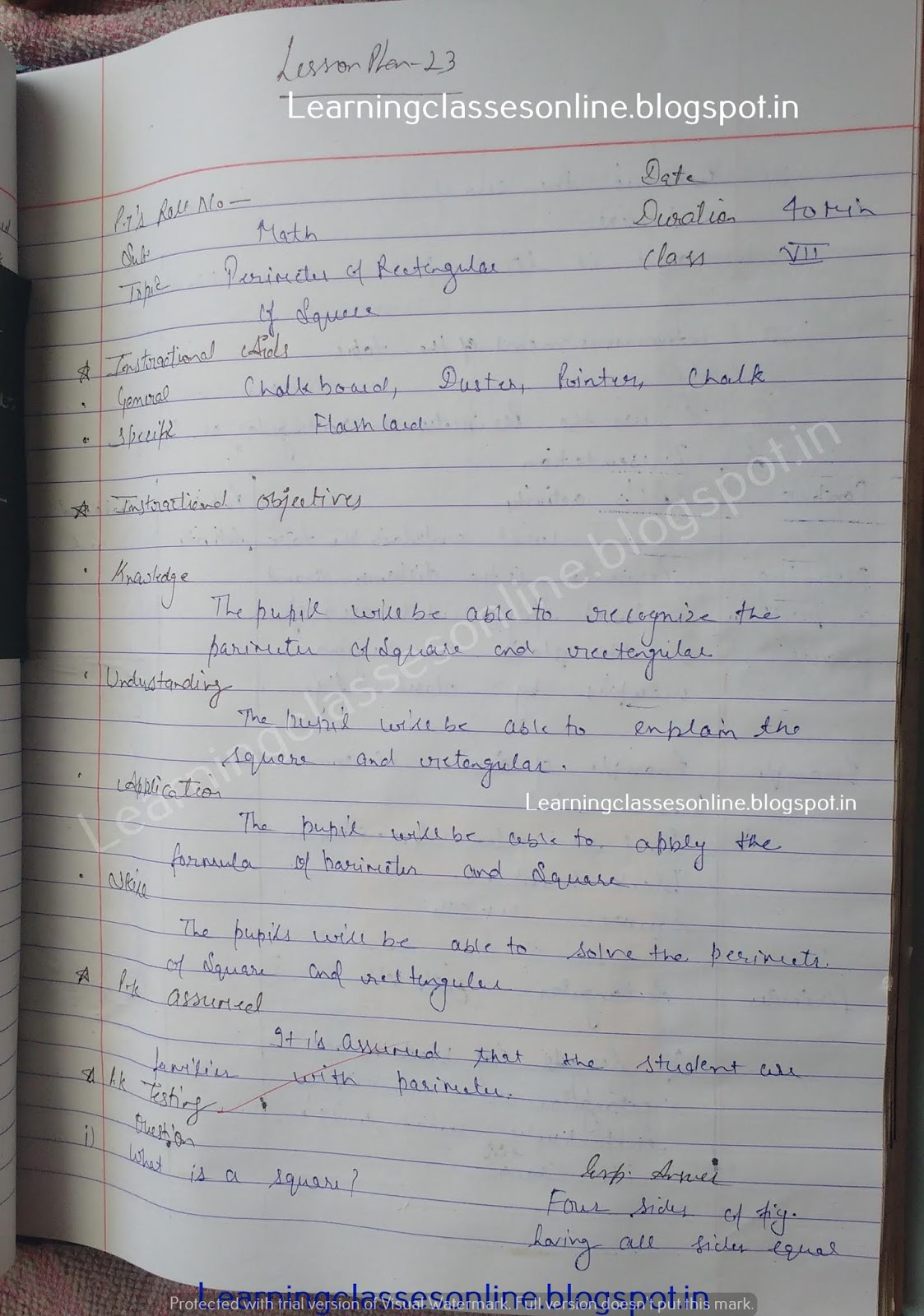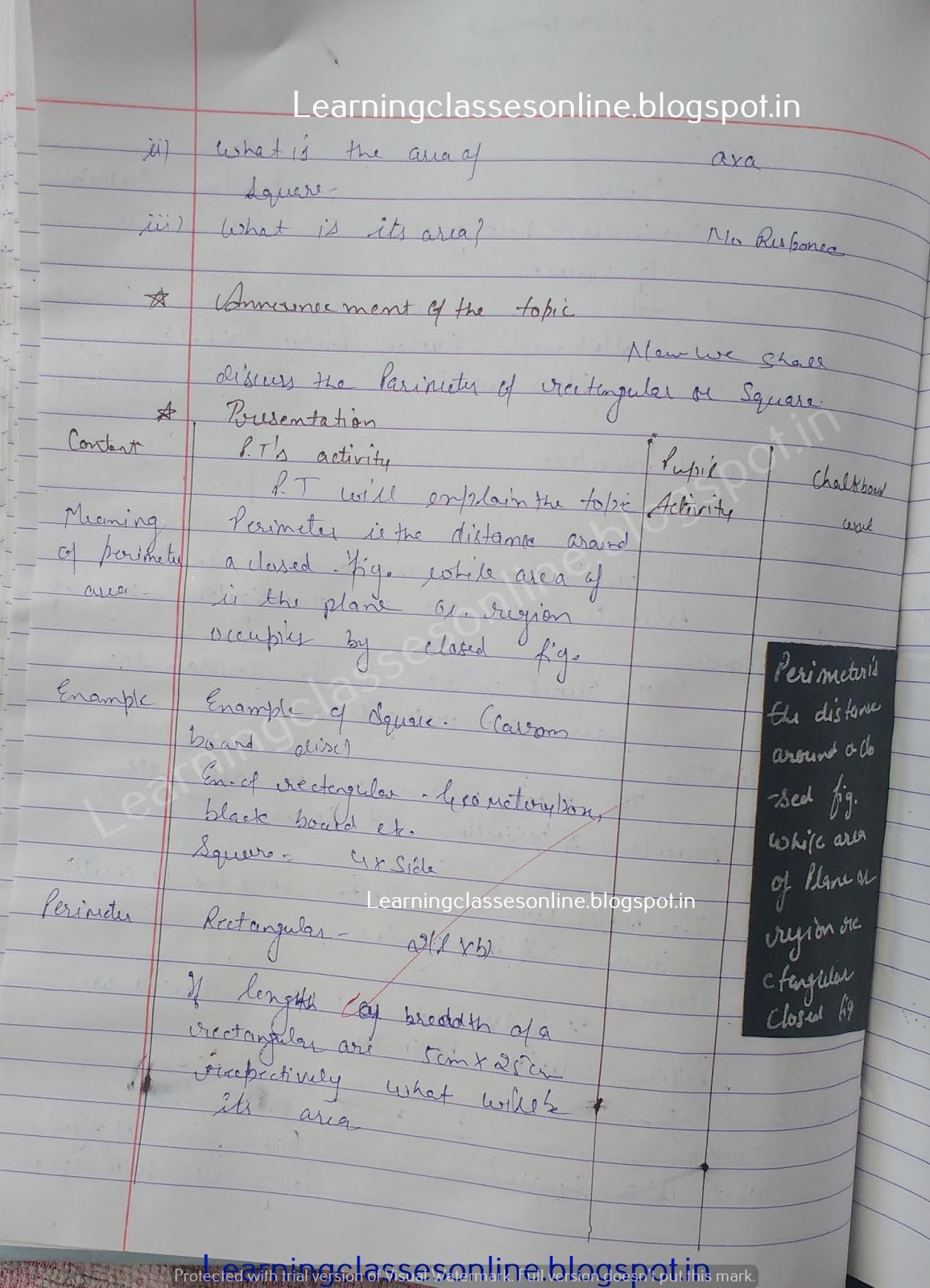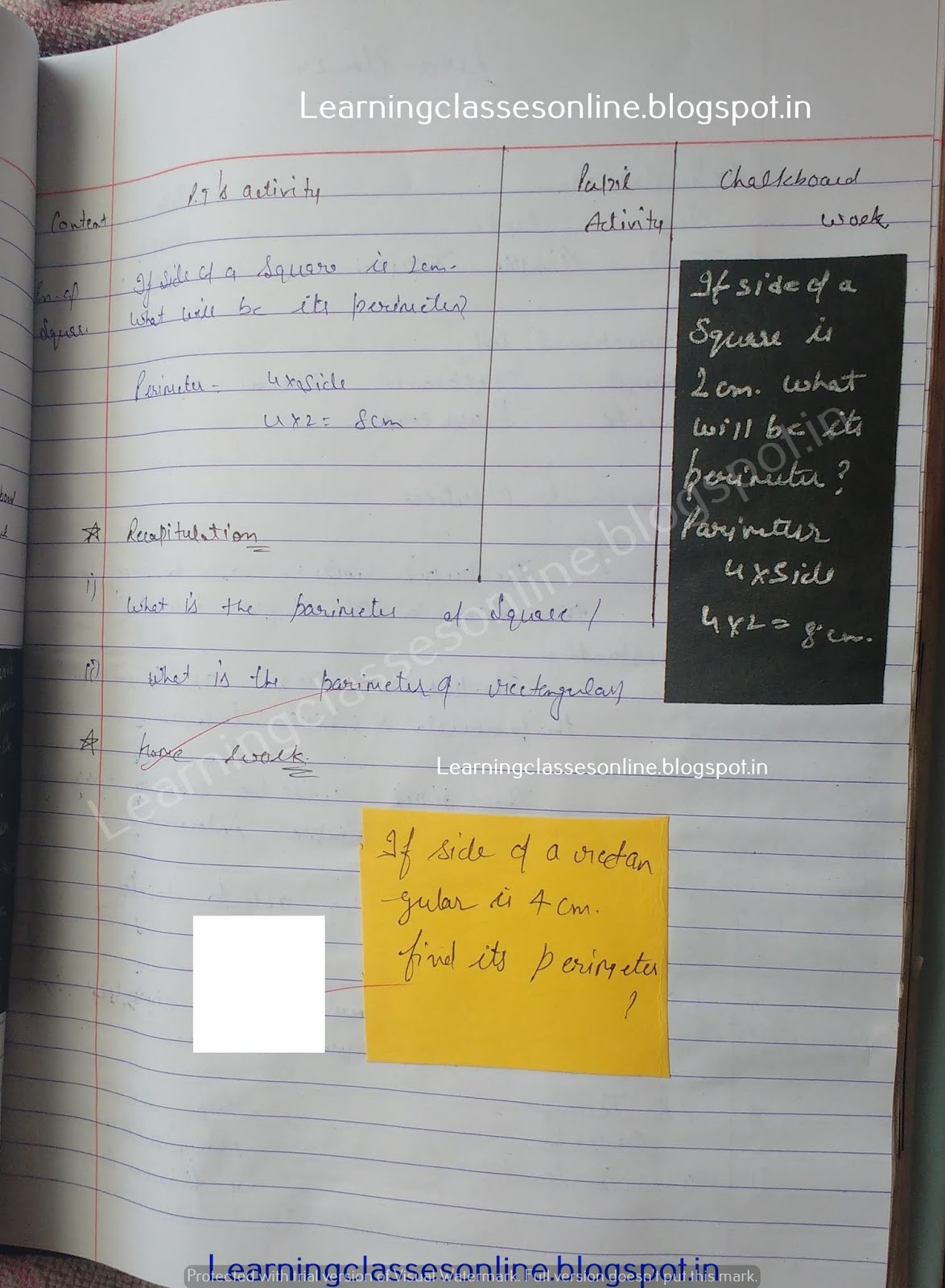Maths Lesson Plan on Perimeter of rectangular of square

# Maths Lesson Plan on Perimeter of rectangular of square

Maths Lesson Plan on Perimeter of rectangular of the square for middle and secondary school teachers.

Note: The Mathematics Lesson Plan given below is just an example. You can change the Name, Class, Course, Date, Duration, etc. according to your needs.Further Reference:
www.learningclassesonline.com Mathematics Lesson Plan for B.Ed